# Rat-race couplers

Click here to go to our main page on couplers and splitters

Click here to go to our page on magic tees (a waveguide network with similar properties to the rat-race)

Click here to go to our page on rat-race versus Gysel couplers

Three-way planar rat-race coupler

Click here to learn about the March coupler, a huge inprovement on the rat-race (new for December 2019)

First, a word on how to spell "rat race". Should it be "rat race", "rat-race", or ratrace? We prefer the first two over the third option but we have seen them all in microwave literature. You can decide for yourself!

Applications of rat-race couplers are numerous, and include mixers and phase shifters. The rat-race gets its name from its circular shape, shown below. The circumference is 1.5 wavelengths. For an equal-split rat-race coupler, the impedance of the entire ring is fixed at 1.41xZ0, or 70.7 ohms for a 50 ohm system. For an input signal Vin, the outputs at ports 2 and 4 (thanks, Tom!) are equal in magnitude, but 180 degrees out of phase.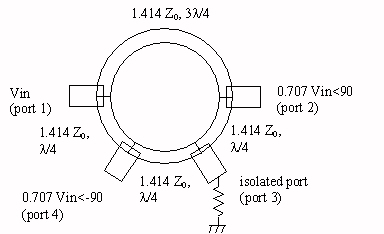Rat-race coupler (equal power split)

Note: Richard from Australia reminded us recently that the rat-race can also be used as an in-phase splitter. If you feed port 4 in the above figure, port 1 and port 3 will receive a 3 dB split, and port 2 will be isolated. This is why port 4 is referred to as the "sum port" and port 1 is referred to as the "delta port", an important consideration in designing monopulse comparators. We'll expand on this idea later. Thanks!

Also, coming soon... a two-stage rat-race design! If you are interested, please This email address is being protected from spambots. You need JavaScript enabled to view it. and we'll try to move this up in the queue.

The coupling of the two arms is shown in the figure below, for an ideal rat-race coupler centered at 10 GHz (10,000 MHz). An equal power split of 3 dB occurs at only the center frequency. The 1-dB bandwidth of the coupled port (S41) is shown by the markers to be 3760 MHz, or 37.6 percent.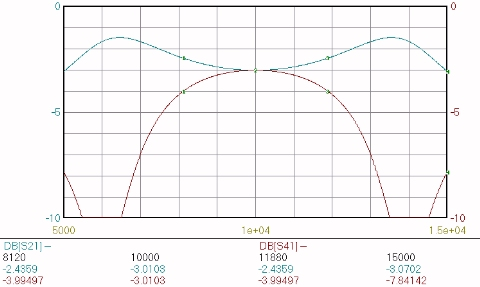Power split of ideal rat-race coupler

The graph below illustrates the impedance match of the same ideal rat-race coupler, at ports 1 and 4. By symmetry, the impedance match at port 3 is the same as at port 1 (S11=S33). For better than 1.5:1 VSWR (14 dB return loss) at all ports (port 4 being the limitation), a bandwidth of 4280 MHz (42.8%) is obtained.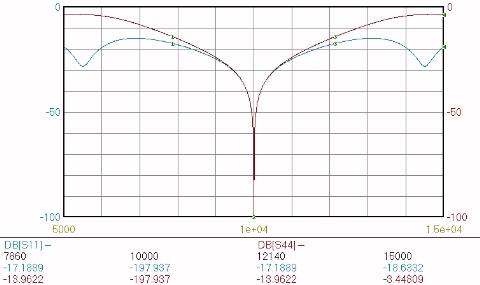Impedance match of ideal rat-race coupler

The next graph shows the isolation between port 1 and port 3 (S31). In the ideal case, it is infinite at the center frequency. The bandwidth over which greater than 20 dB isolation is obtained is 3140 MHz, or 31.4%.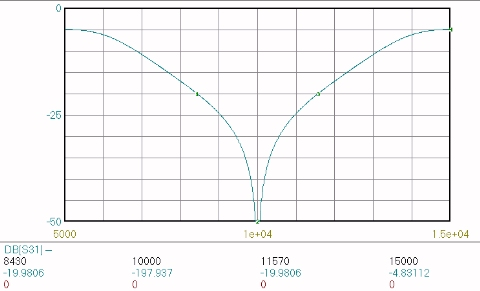Isolation of ideal rat-race coupler

Below the phase difference between arms 2 and 4 is plotted. At the center frequency. a perfect 180 degree difference is observed. The bandwidth that better than +/- 10 degrees is maintained is 3200 MHz, or 32%.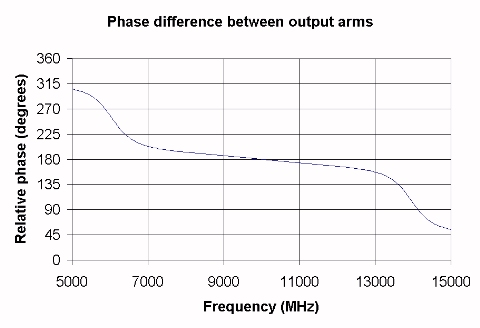### Unequal-split rat-race couplers

In order to provide an unequal split, the impedances of the four arms are varied in pairs, as shown below.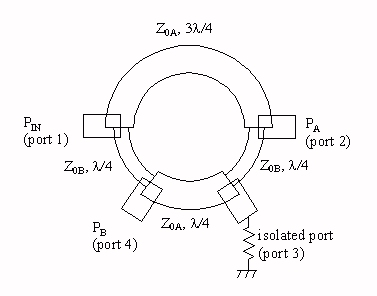Unequal-split rat-race power divider

Equations for the Z0A and Z0B line impedances, as a function of the power split PA/PB, are given below: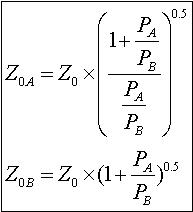Z0A and Z0B are graphed below versus the power split express in dB (coupling ratio) for a 50-0hm system. Click here for info on how to think in dB.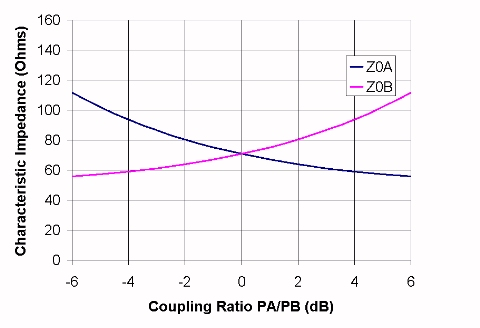The graph below shows the frequency response for a rat-race coupler where PA/PB=0.25. This corresponds to a 50-ohm power divider where the power out of port 2 (PA) is six dB below the power out of port 4 (PB). Solving the above equations for the line impedances yields Z0A=111.6 ohms, and Z0B=55.9 ohms. Note that in many real-life cases, this coupler may prove impractical because a line impedance as high as 111.6 ohms may be difficult to accurately achieve in a 50-ohm system.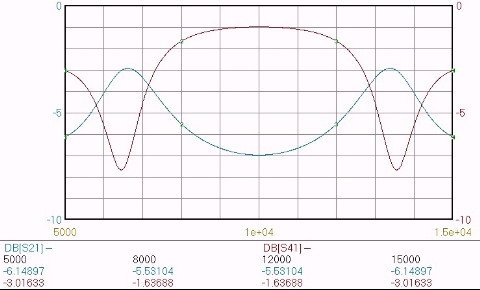Unequal-split rat-race frequency response, PA/PB=0.25

The graph below shows the frequency response for a rat-race coupler where PA/PB=4.0. This corresponds to a power divider where the power out of port 2 (PA)is six dB higher than the power out of port 4 (PB). The line impedances are opposite to the case where PA/PB=0.25; here Z0A=55.9 ohms, and Z0B= 111.6 ohms.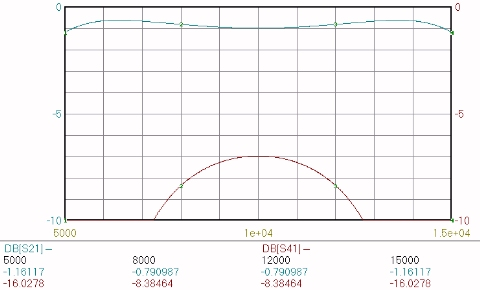Unequal-split rat-race frequency response, PA/PB=4.0

Check out our unequal-split power divider calculator, it handles Wilkinsons, rat-races and branchline couplers.

There's an important degree of freedom that you should know about in rat race couplers. It is described here.

Author : Unknown Editor